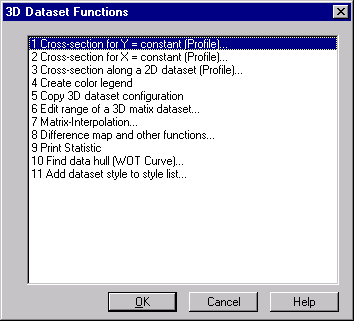# Data=>More Data Functions (3D)¶

In order for this command to be activated, a 3D dataset must be selected.Cross-section for y = constant(Profile), Cross-section for x = constant(Profile)
These functions create a new page with cross sections of the dataset. See Data=>Cross-section along a 2D dataset (Profile) (3D).
Cross-section along a 2D dataset (Profile)
Calculates a cross section value along a 2D dataset. (see Data=>Cross-section (3D)).
Create color legend
Creates a color legend (see Data=>3D Color Legend).
Copy 3D dataset configuration
Copies the dataset configuration to a selected dataset (see Data=>Copy 3D dataset configuration).
Difference map and other functions
Calculates a difference of two maps (see Data=>Difference map and other functions (3D)).
Print Statistic
Creates a Text Object with information about the selected map (see Data=>Statistic (3D)).
Matrix Interpolation
Calculates a new interpolation matrix from the matrix of the selected dataset. (see Data=>Matrix-Interpolation (3D)).
Find data hull (Full load line or WOT)
Finds the full load line of the selected dataset (see Data=>Find data hull (3D)).

Comment

The 3D dataset functions are written in UniScript. The source code is found in the file `rs_xyz.ic`.